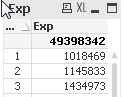QlikView Scripting

Discussion Board for collaboration on QlikView Scripting.

Announcements
QlikWorld 2020: Join us May 11 - 14, 2020 in Phoenix, AZ. Register early and save \$400. Learn More
Highlighted
Contributor

Sum over aggr (in text object)

Dear Community,

i have a straight table with one dimension 'Group' and one expression '=SUM(Aggr(SUM(AMOUNT),GROUP))'

On the top are the totals by expression

The result looks like this :Now i want to display the total 49398... directly inside a text object as a key performance indicator using the same expression

=SUM(Aggr(SUM(AMOUNT),GROUP)). But its not working as the number is not the same comparing with the straight table totalTags (3)
1 Solution

Accepted SolutionsMVP

Re: Sum over aggr (in text object)

maybe this

=sum({\$ <Group*={"*"}>} Amount)

14 RepliesMVP

Re: Sum over aggr

Aggr works with the dimensions inside the chart so try to add all the dimensions used in the chart to your aggr statementPartner

Re: Sum over aggr

you need to ignore dimension by using total

ex:sum(total column)

I can walk on water when it freezes
Contributor

Re: Sum over aggr (in text object)

Inside the straight table we have only one dimension called 'Group', so the total value should be the same inside the table and text object

Contributor

Re: Sum over aggr (in text object)

I tried to use =SUM(Aggr(SUM(Total AMOUNT),GROUP)) but the value was also wrongMVP

Re: Sum over aggr (in text object)

Could you please try with the following expession in the chart and in the text box

'=SUM(Aggr(NODISTINCT SUM(AMOUNT),GROUP))'Partner

Re: Sum over aggr (in text object)

may you please supply a sample QVW ?

I can walk on water when it freezesMVP

Re: Sum over aggr (in text object)

This expression in chart with Group as a dimension

=SUM(Aggr(SUM(AMOUNT),GROUP))

is just an inefficient way of saying

=SUM(AMOUNT)

The first expression is the correct way of expressing the sum and is the equivalent of the chart. If the totals are different, then you are using an expression total rather than a sum of rows, and the equivalent would then be the second expression. Unless you have some restrictions in the chart such as a calculated dimension that filters GROUP.

If that does not resolve the problem, then I suggest you post your qvw or a representative sample to see why it is behaving this way.

Logic will get you from a to b. Imagination will take you everywhere. - A Einstein
Contributor

Re: Sum over aggr (in text object)

I have prepared an example qvw.file please see attached.

Contributor

Re: Sum over aggr (in text object)

See attachement below